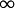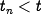Errata: In equation (5.5), the upper limit of the integral should be t

In equation (5.5), the upper limit on the integral should beinstead of:Related to this error, the summation in the previous displayed equation should be modified so that, otherwise the sum could include inputs that are beyond the current time. Hence the displayed equation on lines 5-8 should read